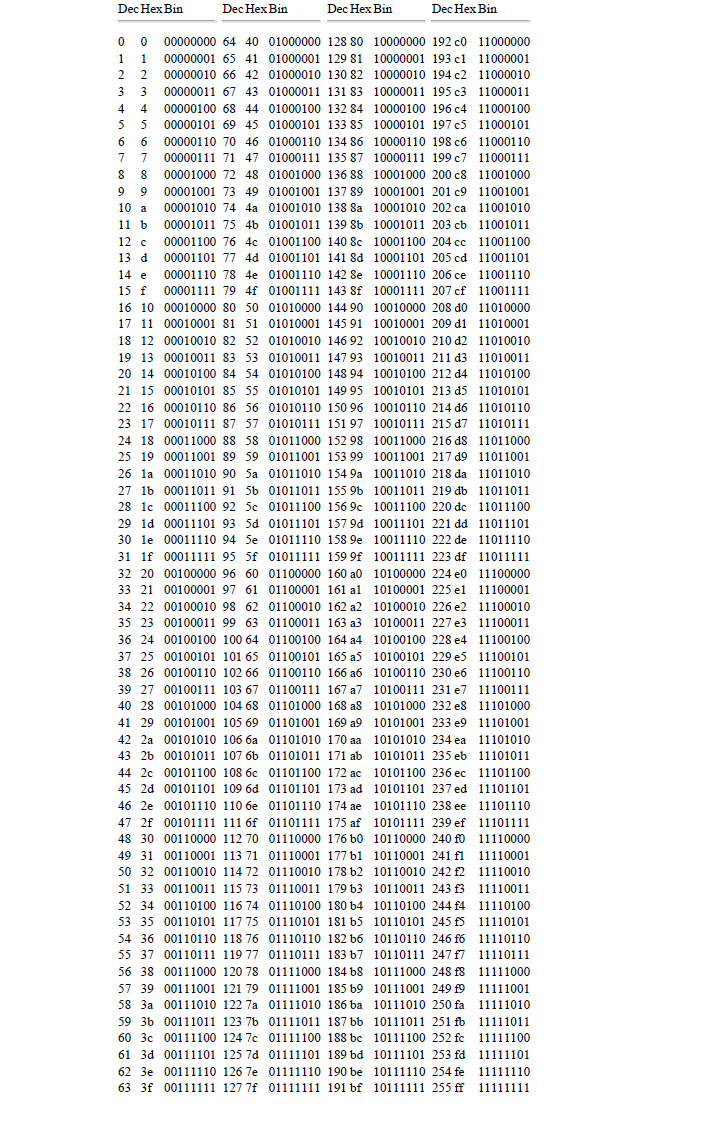# Conversion Table Decimal – Hexadecimal – Binary

Below is a complete table that can help you understand the relation between decimal to binary and binary to decimal conversion.

This table also includes the equivalent values of hexadecimal numbers.

You can search our website for more detail on this topic, we have plenty of pages that cover the number system conversions.The decimal number system is fine for calculations done by humans, but it is not the easiest system for a computer to use. A digital computer contains elements that can be in either of two states: on or off, magnetized or not magnetized, and so on.

For such devices, calculations are most conveniently done using binary numbers. In this chapter we learn what binary numbers are and how to convert between binary and decimal numbers.

Binary numbers are useful in a computer, where each binary digit (bit) can be represented by one state of a “binary switch” that is either on or off.

However, binary numbers are hard to read, partly because of their great length. To represent a nine-digit Social Security number, for example, requires a binary number 29 bits long.

So, in addition to binary numbers, we also study other ways in which numbers can be represented. Hexadecimal, octal, and binary-coded decimal systems allow us to express binary numbers more compactly, and they make the transfer of data between computers and people much easier. Here we learn how to convert numbers between each of these systems, and between decimal and binary as well.

## Bits, Bytes, and Words

Each of the digits is called a bit, from binary digit. A byte is a group of 8 bits, and a word is the largest string of bits that a computer can handle in one operation.

The number of bits in a word is called the word length.

Different computers have different word lengths, with 8, 16, or 32 bits being common for desktop or personal computers.

The longer words are often broken down into bytes for easier handling.

Half a byte (4 bits) is called a nibble. A kilobyte (Kbyte or KB) is 1024 (210) bytes, and a megabyte (Mbyte or MB) is 1,048,575 (220) bytes.

error: Content is protected !!